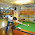### Minimum sum of numbers in an array

Source: Asked to me on quora ( cseblog.quora.com )

Problem:

Given an array of n positive numbers (n ~ 100000), what is the algorithmic approach to find the minimum possible sum (>=0) by using all the numbers in an array?

Example 1:
1 2 2 3 4

Example 2:
2 3 4 7 13

1.Since the array is large and unsorted (assumption), I think a greedy approach with backtracking is advisable.

Mohan S N

2.Assuming that the numbers are integers, this problem can be solved using integer knapsack problem. Let the sum of all the numbers in the array be S. Think of a bag of size S/2. The problem now becomes making a subset from the array having sum less than or equal to S/2. Just be careful while obtaining S, as it might be larger than maximum allowed value for a positive integer. Use a proper database to hold that.

1.3.At every iteration, find the largest 2 integers in the array ('n' steps), remove and subtract them, and insert the difference back to the array. Do this 'n' times. We have the result in O(n^2) time.

1.2.Consider the following example 3,3,3,7,8, 8
optimal is 3+3+3+7-8-8 =0
But your method finds the optimal in the set 3,3,3,7,0 which is not 0 clearly.

So the method don't work if the largest 2 integers belong to same category

4.Are we over estimating this problem ?

5.i dont get the problem ... in example 1 where is -2 ?? all are positive numberz??

6.Create the max heap (O(n)). Maintain two running counters. Take the first number from heap and add it to counter1, take the 2nd number form the heap and add it to counter2. After that, take the next number from the heap and add it to the counter that minimizes |counter1 - counter2|, i.e., always add that number to the counter that is currently smaller of the two. Keep doing this and you will get the answer in O(n log n). Proof: Is by induction...

7.Recursive Approach (inefficient though(2^n complexity) but I Like Recursion!):
int minsum(int* arr,int n, int m)//n is number of elements left to consider, m is the min sum
{
if(n==1)
{
if *arr>=m return *arr;
else return infinity;
int a=minsum(arr+1,n-1,m-*arr);
int b=minsum(arr+1,n-1,m+*arr);
return(a+*arr<b-*arr?a+*arr:b-*arr);
}
in main call minsum(arr,n,0)